# 1. random伪随机数生成器

random模块基于Mersenne Twister算法提供了一个快速伪随机数生成器。原来开发这个生成器是为了向蒙特卡洛模拟生成输入，Mersenne Twister算法会生成大周期近均匀分布的数，因此适用于大量不同类型的应用。

## 1.1 生成随机数

random()函数从所生成的序列返回下一个随机的浮点值。返回的所有值都落在0<=n<1.0区间内。

import random

for i in range(5):
print('%04.3f' % random.random(), end=' ')
print()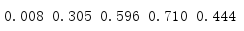import random

for i in range(5):
print('{:04.3f}'.format(random.uniform(1, 100)), end=' ')
print()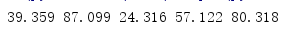## 1.2 指定种子

import random

random.seed(1)

for i in range(5):
print('{:04.3f}'.format(random.random()), end=' ')
print()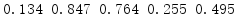## 1.3 保存状态

random()使用的伪随机算法的内部状态可以保存，并用于控制后续生成的随机数。如果在继续生成随机数之前恢复前一个状态，则会减少出现重复的可能性，即避免出现之前输入中重复的值或值序列。getstate()函数会返回一些数据，以后可以借助setstate()利用这些数据重新初始化伪随机数生成器。

import random
import os
import pickle

if os.path.exists('state.dat'):
# Restore the previously saved state
print('Found state.dat, initializing random module')
with open('state.dat', 'rb') as f:
random.setstate(state)
else:
# Use a well-known start state
print('No state.dat, seeding')
random.seed(1)

# Produce random values
for i in range(3):
print('{:04.3f}'.format(random.random()), end=' ')
print()

# Save state for next time
with open('state.dat', 'wb') as f:
pickle.dump(random.getstate(), f)

# Produce more random values
print('\nAfter saving state:')
for i in range(3):
print('{:04.3f}'.format(random.random()), end=' ')
print()

getstate()返回的数据是一个实现细节，所以这个例子用pickle将数据保存到一个文件；否则，它会把伪随机数生成器当作一个黑盒。如果程序开始时这个文件存在，则加载原来的状态并继续。每次运行时都会在保存状态之前和之后生成一些数，以展示恢复状态会使生成器再次生成同样的值。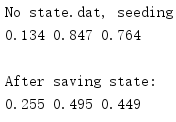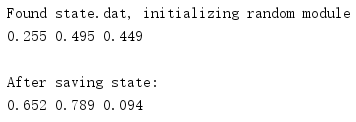## 1.4 随机整数

random()将生成浮点数。可以把结果转换为整数，不过直接使用randint()生成整数会更方便。

import random

print('[1, 100]:', end=' ')

for i in range(3):
print(random.randint(1, 100), end=' ')

print('\n[-5, 5]:', end=' ')
for i in range(3):
print(random.randint(-5, 5), end=' ')
print()

randint()的参数是值的闭区间的两端。这些数可以是正数或负数，不过第一个值要小于第二个值。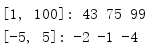randrange()是从区间选择值的一种更一般的形式。

import random

for i in range(3):
print(random.randrange(0, 101, 5), end=' ')
print()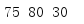## 1.5 选择随机元素

import random
import itertools

outcomes = {
'tails': 0,
}
sides = list(outcomes.keys())

for i in range(10000):
outcomes[random.choice(sides)] += 1

print('Tails:', outcomes['tails'])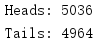## 1.6 排列

import random
import itertools

FACE_CARDS = ('J', 'Q', 'K', 'A')
SUITS = ('H', 'D', 'C', 'S')

def new_deck():
return [
# Always use 2 places for the value, so the strings
# are a consistent width.
'{:>2}{}'.format(*c)
for c in itertools.product(
itertools.chain(range(2, 11), FACE_CARDS),
SUITS,
)
]

def show_deck(deck):
p_deck = deck[:]
while p_deck:
row = p_deck[:13]
p_deck = p_deck[13:]
for j in row:
print(j, end=' ')
print()

# Make a new deck, with the cards in order
deck = new_deck()
print('Initial deck:')
show_deck(deck)

# Shuffle the deck to randomize the order
random.shuffle(deck)
print('\nShuffled deck:')
show_deck(deck)

# Deal 4 hands of 5 cards each
hands = [[], [], [], []]

for i in range(5):
for h in hands:
h.append(deck.pop())

# Show the hands
print('\nHands:')
for n, h in enumerate(hands):
print('{}:'.format(n + 1), end=' ')
for c in h:
print(c, end=' ')
print()

# Show the remaining deck
print('\nRemaining deck:')
show_deck(deck)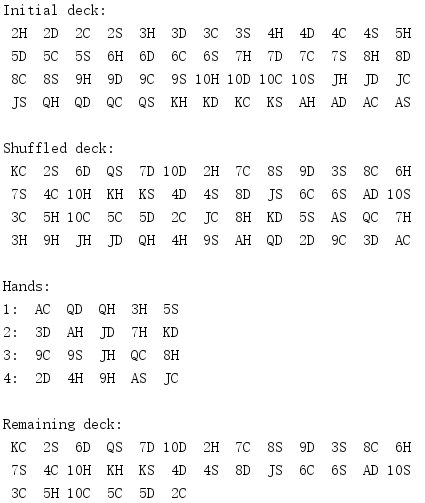## 1.7 采样

words.txt

pear
apricot
grape
pineapple
apple
peach
banana
plum
watermelon
lemon
orange
mango
strawberry

Demo.py

import random

with open('words.txt', 'rt') as f:
words = [w.rstrip() for w in words]

for w in random.sample(words, 5):
print(w)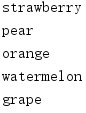## 1.8 多个并发生成器

import random
import time

print('Default initializiation:\n')

r1 = random.Random()
r2 = random.Random()

for i in range(3):
print('{:04.3f}  {:04.3f}'.format(r1.random(), r2.random()))

print('\nSame seed:\n')

seed = time.time()
r1 = random.Random(seed)
r2 = random.Random(seed)

for i in range(3):
print('{:04.3f}  {:04.3f}'.format(r1.random(), r2.random()))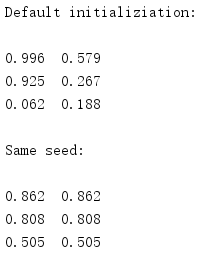## 1.9 SystemRandom

import random
import time

print('Default initializiation:\n')

r1 = random.SystemRandom()
r2 = random.SystemRandom()

for i in range(3):
print('{:04.3f}  {:04.3f}'.format(r1.random(), r2.random()))

print('\nSame seed:\n')

seed = time.time()
r1 = random.SystemRandom(seed)
r2 = random.SystemRandom(seed)

for i in range(3):
print('{:04.3f}  {:04.3f}'.format(r1.random(), r2.random()))

SystemRandom产生的序列是不可再生的，因为其随机性来自系统，而不是来自软件状态(实际上，seed()和setstate()根本不起作用)。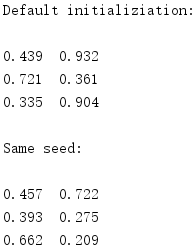posted @ 2020-03-07 08:55  SmallGrayCode  阅读(1327)  评论(0编辑  收藏  举报